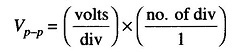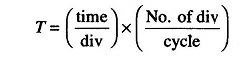## Applications of Oscilloscope:

The range of applications of oscilloscope varies from basic voltage measurements and waveform observation to highly specialized applications in all areas of science, engineering and technology.

### Voltage Measurements:

The most direct voltage measurement made with the help of an oscilloscope is the peak to peak (p-p) value. The rms value of the voltage can then be easily calculated from the p-p value.

To measure the voltage from the CRT display, one must observe the setting of the vertical attenuator expressed in V/div and the peak to peak deflection of the beam, i.e. the number of divisions. The peak to peak value of voltage is then computed as follows.### Period and Frequency Measurements:

Another applications of oscilloscope is the period and frequency measurements. The period and frequency of periodic signals are easily measured with an oscilloscope. The waveform must be displayed such that a complete cycle is displayed on the CRT screen. Accuracy is generally improved if a single cycle displayed fills as much of the horizontal distance across the screen as possible.

The period is calculated as follows.The frequency is then calculated as f = 1/T.

Scroll to Top